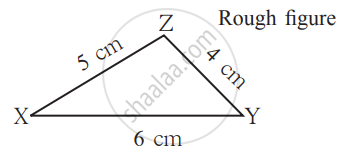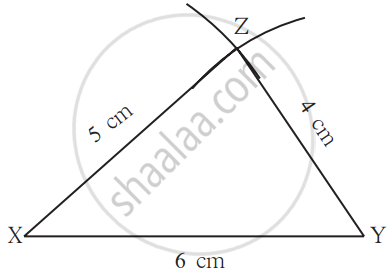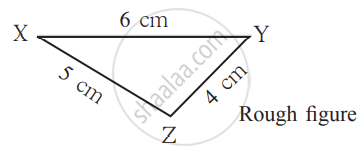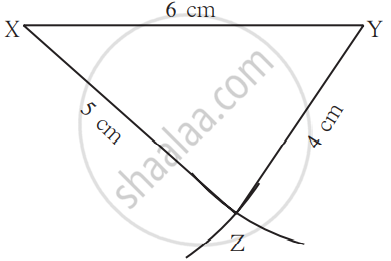# Construct a triangle given the lengths of its three sides:

1) Draw ΔXYZ such that l(XY) = 6 cm, l(YZ) = 4 cm, l(XZ) = 5 cm.

Let us draw a rough figure quickly and show the given information in it as accurately as possible. For example, side XY is the longest, so, in the rough figure, too, XY should be the longest side.

## Steps:

1. According to the rough figure, segment XY of length 6 cm is drawn as the base.2. As l(XZ) is 5 cm, draw an arc on one side of seg XY with the compass opened to 5 cm and with its point at X.

3. Next, with the point at Y and the compass opened to 4 cm, draw an arc to cut the first arc at Z. Draw segs XY and YZ.4. A similar construction can be drawn on the other side of the base as shown below.If you would like to contribute notes or other learning material, please submit them using the button below.

### Shaalaa.com

Construct a Triangle Given the Lengths of Its Three Sides [00:02:00]
S
0%